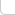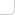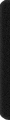# 某岛

… : "…アッカリ～ン . .. . " .. .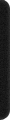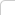June 27, 2013

# HDU 2871. Memory Control

### Brief description:

Memory units are numbered from 1 up to N. A sequence of memory units is called a memory block.
The memory control system we consider now has four kinds of operations:

1. `Reset`: Reset all memory units free. For each `Reset` operation, output `Reset Now`.
2. `New x`: Allocate a memory block consisted of x continuous free memory units with the least start number. For each `New` operation, if it’s possible to allocate a memory block, output `New at A`, where A is the least start number, otherwise output `Reject New`.
3. `Free x`: Release the memory block which includes unit x. For each `Free` operation, if it’s possible to find a memory block occupy unit x, output `Free from A to B`, where A and B refer to the start and end number of the memory block, otherwise output `Reject Free`.
4. `Get x`: Return the start number of the xth memory block(Note that we count the memory blocks allocated from left to right). For each `Get` operation, if it’s possible to find the xth memory blocks, output `Get at A`, where A is its start number, otherwise output `Reject Get`.
5. Where 1<=x<=N. You are request to find out the output for M operations. Output one blank line after each test case.

### Analysis: